DATA MINING
Desktop Survival Guide
by Graham Williams### Alternating Decision Tree

An alternating decision tree (), combines the simplicity of a single decision tree with the effectiveness of boosting. The knowledge representation combines tree stumps, a common model deployed in boosting, into a decision tree type structure. The different branches are no longer mutually exclusive. The root node is a prediction node, and has just a numeric score. The next layer of nodes are decision nodes, and are essentially a collection of decision tree stumps. The next layer then consists of prediction nodes, and so on, alternating between prediction nodes and decision nodes.

A model is deployed by identifying the possibly multiple paths from the root node to the leaves through the alternating decision tree that correspond to the values for the variables of an observation to be classified. The observation's classification score (or measure of confidence) is the sum of the prediction values along the corresponding paths. A simple example involving the variables Income and Deduction (with values \$56,378, and \$1,429, respectively), will result in a score of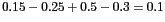. This is a positive number so we will place this observation into the positive class, with a confidence of only 0.1. The corresponding paths in Figure  is highlighted.

We can build an alternating decision tree in R using the RWeka package:

 ```# Load the sample dataset > data(audit, package="rattle") # Load the RWeka library > library(RWeka) # Create interface to Weka's ADTree and print some documentation > ADT <- make_Weka_classifier("weka/classifiers/trees/ADTree") > ADT > WOW(ADT) # Create a training subset > set.seed(123) > trainset <- sample(nrow(audit), 1400) # Build the model > audit.adt <- ADT(as.factor(Adjusted) ~ ., data=audit[trainset, c(2:11,13)]) > audit.adt Alternating decision tree: : -0.568 | (1)Marital = Married: 0.476 | | (10)Occupation = Executive: 0.481 | | (10)Occupation != Executive: -0.074 | (1)Marital != Married: -0.764 | | (2)Age < 26.5: -1.312 | | | (6)Marital = Unmarried: 1.235 | | | (6)Marital != Unmarried: -1.366 | | (2)Age >= 26.5: 0.213 | (3)Deductions < 1561.667: -0.055 | (3)Deductions >= 1561.667: 1.774 | (4)Education = Bachelor: 0.455 | (4)Education != Bachelor: -0.126 | (5)Occupation = Service: -0.953 | (5)Occupation != Service: 0.052 | | (7)Hours < 49.5: -0.138 | | | (9)Education = Master: 0.878 | | | (9)Education != Master: -0.075 | | (7)Hours >= 49.5: 0.339 | (8)Age < 36.5: -0.298 | (8)Age >= 36.5: 0.153 Legend: -ve = 0, +ve = 1 Tree size (total number of nodes): 31 Leaves (number of predictor nodes): 21 ```

We can pictorially present the resulting model as in Figure 14.1, which shows a cut down version of the actual ADTree built above. We can explore exactly how the model works using the simpler model in Figure 14.1. We begin with a new instance and a starting score of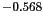. Suppose the person is married and aged 32. Considering the right branch we add. We also consider the left branch, we add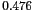. Supposing that they are not an Executive, we add another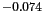. The final score is then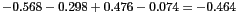.

 ```# Explore the results > predict(audit.adt, audit[-trainset, -12]) > predict(audit.adt, audit[-trainset, -12], type="prob") # Plot the results > pr <- predict(audit.adt, audit[-trainset, c(2:11,13)], type="prob")[,2] > eval <- evaluateRisk(pr, audit[-trainset, c(2:11,13)]\$Adjusted, audit[-trainset, c(2:11,13,12)]\$Adjustment) > title(main="Risk Chart ADT audit [test] Adjustment", sub=paste("Rattle", Sys.time(), Sys.info()["user"])) ```